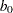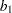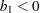# Create a Title Based on an Analysis

This section adds a title to the graph that shows the fitted model. You can add a title by calling the SetTitleText and ShowTitle methods of the Plot class. However, in this case you first need to convert the values stored in the Estimate matrix from numerical to character values. One way to do this is to apply a w.d format by using the Base SAS function PUTN. You can then concatenate pieces of the title together using the SAS/IML CONCAT function and display the result.

The statements that follow build the title intelligently. Letbe the intercept andbe the slope of the regression line. The regression line for this example has negative slope (). If you built the title as

```   title = concat("wind_kts = ", b0, " + ", b1, " * min_pressure");
```

then the title string might appear as

```   wind_kts = 1333.35 + -1.29 * min_pressure
```

While this is correct, it is awkward to read. A more aesthetic title would be

```   wind_kts = 1333.35 - 1.29 * min_pressure
```

This can be accomplished by treating the case of negative slope separately from the case of positive slope.

Add the following statements at the bottom of the program window, and select ProgramRun from the main menu. Figure 8.2 shows how the title appears.

```   b0 = putn( Estimate, "7.2" );
if Estimate<0 then do;          /* if slope is negative */
sign = " - ";                   /* display b0 - (-b1)   */
b1 = putn( -Estimate, "4.2" );
end;
else do;                           /* else display b0 + b1 */
sign = " + ";
b1 = putn( Estimate, "4.2" );
end;
title = concat( "wind_kts = ", b0, sign, b1, " * min_pressure" );
FitPlot.SetTitleText( title );
FitPlot.ShowTitle();
```Previous Page | Next Page | Top of Page